| Content Writer: Poornachandra Sarang | Technical Review: Aaditya Damle and ABCOM Team | Copy Editor: Anushka Devasthale | Level: Advanced | Banner Image Source : Internet |Image Source: Internet

Imagine yourself in the shoes of a site administrator of a large content production company. Your site allows the readers to post their feedback on the articles posted on your site regularly. Such reviews' daily intakes may be extremely high, and somebody needs to control the toxicity (the slang, abuses) of these comments before others get at it. In short, you need to have a mechanism to monitor this toxicity in real-time. Here is where the present-day NLP (Natural Language Processing) models come in play to save your day!

In this tutorial, you will learn how to use the most talked about NLP model - BERT (Bidirectional Encoder Representations for Transformers) to detect the toxicity in a given text para.

## Project Description

Dataset is the fuel to run any ML project. We need a Dataset having all the toxic words marked out for our project related to toxicity detection. Fortunately, Kaggle held a competition two years ago called the Toxic Comment Classification Challenge to identify and classify toxic comments. We will be using the dataset that was provided for the competition. You will need to download and use this dataset for developing your toxic content detection model.

This problem-statement is essentially a text classification problem, and you will be using BERT for this purpose. The model will predict how much toxicity the given passage contains.

Here is a little more insight into BERT; it was created and published in 2018 by Jacob Devlin and his colleagues working at Google. Google is leveraging BERT to understand user searches better. BERT has given state-of-the-art results on a wide variety of natural language processing tasks such as text classification, sentiment analysis, question answering, etc. Bert was pre-trained on Wikipedia (2500 million words) and BookCorpus (800 million words). With task-specific fine-tuning, it can be used for a wide variety of NLP applications.

BERT is a bunch of Transformers and encoders stacked together. It is bidirectional as it performs self-attention in both directions. It has 12 transformer blocks and 12 attention blocks (110 trainable parameters). BERT has large 24 blocks and 16 attention heads (340 million endpoints). BERT has inspired many modern NLP architectures and language models, such as Google’s TransformerXL, OpenAI’s GPT-2, XLNet, ERNIE2.0, etc.

In this project, we will be making the most out of this pre-trained model for encoding our text and then detecting toxicity in the given text.

## Creating a Project

Follow along and create a new Google Colab project and rename it to ToxicCommentDetector. If you are unfamiliar with Colab, here is a short tutorial to get you started.

### Import Libraries

You will use the Transformers library by Hugging Face. The Hugging Face transformers package is an immensely popular Python library providing pre-trained models that are extraordinarily useful for various Natural Language Processing (NLP) tasks. To install this library, use the following pip command.

``````!pip install transformers
``````

Import the other required libraries:

``````import os
import numpy as np
import pandas as pd
import tensorflow as tf
tf.compat.v1.logging.set_verbosity(tf.compat.v1.logging.ERROR)
from tokenizers import BertWordPieceTokenizer
from tqdm.notebook import tqdm
from tensorflow.keras.layers import Dense, Input
from tensorflow.keras.models import Model
from tensorflow.keras.callbacks import ModelCheckpoint
from tensorflow.keras import backend as K
import transformers
from transformers import TFAutoModel, AutoTokenizer
import matplotlib.pyplot as plt
``````

### Checking for TPU

As using BERT requires heavy processing, using TPU is recommended instead. You can opt for the TPU usage by selecting the Runtime/Change runtime type, menu option in your project.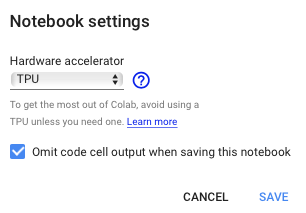Google requests you to use TPU judiciously, so do not use it as a default for all your Colab projects. You can check if TPU is available for you and if yes then set the distribution strategy using the following code:

``````try:
tpu = tf.distribute.cluster_resolver.TPUClusterResolver()
print('Running on TPU ', tpu.master())
except ValueError:
tpu = None

if tpu:
tf.config.experimental_connect_to_cluster(tpu)
tf.tpu.experimental.initialize_tpu_system(tpu)
strategy = tf.distribute.experimental.TPUStrategy(tpu)
else:
strategy = tf.distribute.get_strategy()
``````

TPUs are usually on-cloud TPU workers and require some initialization to connect to the remote cluster. The TPU argument to TPUClusterResolver in the above code is an exclusive address just for the Colab. In case you are running the project on Google Compute Engine (GCE), you should instead pass in the name of your CloudTPU. A distribution strategy in the above code is an abstraction used to drive models on CPUs, GPUs, or TPUs. Use the appropriate distribution strategy to run the model on the desired device.

That is all our prerequisites; let us download and study the dataset before building the model.

For your quick use, you can download the dataset from our git repository using wget as follows:

``````!wget https://raw.githubusercontent.com/abcom-mltutorials/detecting-slang/master/jigsawdata.zip
``````

``````!unzip '/content/jigsawdata.zip'
``````

This command will create the following folders and files will on your drive.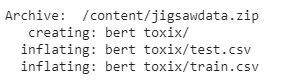Kaggle has provided the training and testing data in two separate folders. Load the two CSV files in the project using the Pandas data read method.

``````train = pd.read_csv("/content/bert toxix/train.csv")
``````

Try printing records from the training set containing toxic contents:

``````train[train['toxic']==1]
``````

The output will be as follows;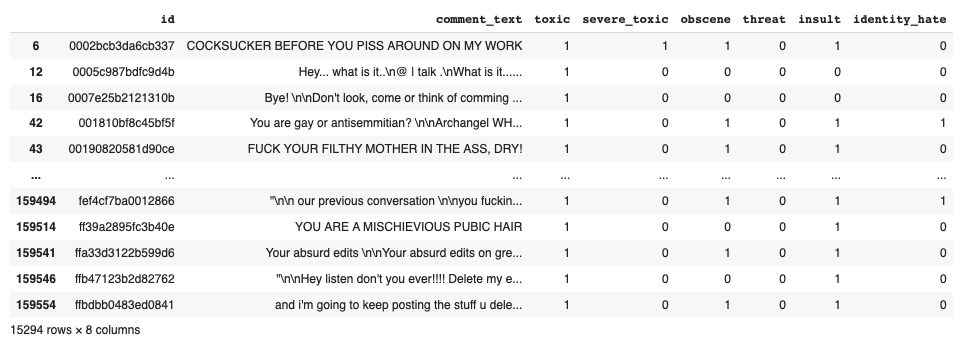The dataset consists of 15294 rows and eight columns. Each row contains the text and absence/presence of slang words.

You can look at the distribution of target values in the entire dataset with the following code:

``````columns=['toxic','severe_toxic','obscene','threat','insult','identity_hate']
zeros =[]
ones =[]
for col in columns:
zeros.append(train[col].value_counts())
ones.append(train[col].value_counts())

df = pd.DataFrame({'zero': zeros,'one': ones}, index=columns)
df.plot.bar(rot=0)
``````

The output is as follows: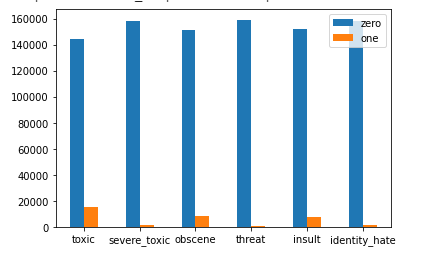Next, we will now build our model based on BERT.

### Building Keras Model

To build the model, we define a function called `build_model` as follows:

``````def build_model(transformer, loss, max_len=512):
input_word_ids = Input(shape=(max_len,), dtype=tf.int32, name="input_word_ids")
sequence_output = transformer(input_word_ids)
cls_token = sequence_output[:, 0, :]
x = tf.keras.layers.Dropout(0.35)(cls_token)
out = Dense(1, activation='sigmoid')(x)

model = Model(inputs=input_word_ids, outputs=out)

return model
``````

The build method receives an instance of a pre-trained BERT model in its first parameter called a transformer. The second parameter specifies the loss function to be used.

We passed the loss function as a parameter here so that we can experiment with different loss functions. Upon doing this, I found that instead of using the traditional `binary_crossentropy`, the new Focal loss function gives better results. We will discuss the focal loss function in the following section.

The first layer is the input layer, which takes an input of `max_len`. Note that we will pad all our input sentences to this length. The transformer output is passed through a dropout layer and finally through a sigmoid activated dense layer that gives us a binary output. The output would indicate whether the input is toxic or not. The model is compiled with Adam optimizer and the Focal loss function.

### The Focal Loss Function

The newly introduced focal loss function is created specifically to deal with the data imbalance problem for one-staged detectors. It improves training. You can read more about it in the references cited at the end of the tutorial. The function definition is given below:

``````def focal_loss(gamma=2., alpha=.2):
def focal_loss_fixed(y_true, y_pred):
pt_1 = tf.where(tf.equal(y_true, 1), y_pred, tf.ones_like(y_pred))
pt_0 = tf.where(tf.equal(y_true, 0), y_pred, tf.zeros_like(y_pred))
return -K.mean(alpha *
K.pow(1. - pt_1, gamma) *
K.log(pt_1)) - K.mean((1 - alpha) *
K.pow(pt_0, gamma) *
K.log(1. - pt_0))
return focal_loss_fixed
``````

### Instantiating Model

We instantiate our model by first creating an instance of the pre-trained BERT model and then passing it to our build_model method.

``````with strategy.scope():
transformer_layer = transformers.TFBertModel.from_pretrained('bert-base-uncased')
model = build_model(transformer_layer, loss=focal_loss(gamma=1.5), max_len=512)
model.summary()
``````

The output summary is shown below: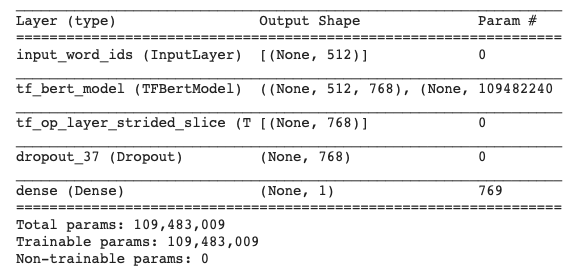As you can see, we have an input layer feeding data into the BERT model and a dense output layer giving us the binary output. So the model architecture is quite trivial. Most of the processing is done in the BERT model, and its entire architecture is hidden in our model’s architecture.

Now, let us start preparing the data for feeding it to the above-defined model.

### Data Preprocessing

We first tokenize all our input datasets - training and testing. You will use the BERT pre-trained tokenizer for this purpose. You create a tokenizer instance using the following statement:

``````tokenizer = transformers.BertTokenizer.from_pretrained('bert-base-uncased')
``````

``````save_path = 'distilbert_base_uncased/'
if not os.path.exists(save_path):
os.makedirs(save_path)
tokenizer.save_pretrained(save_path)
``````

Reload it with the Hugging Face tokenizers library

``````fast_tokenizer = BertWordPieceTokenizer('distilbert_base_uncased/vocab.txt', lowercase=True)
fast_tokenizer
``````

You will be using this fast_tokenizer to encode our input. Next, we write an Encode function that uses the above tokenizer to encode the given text.

``````def fast_encode(texts, tokenizer, chunk_size=256, maxlen=512):
tokenizer.enable_truncation(max_length=maxlen)
all_ids = []

for i in tqdm(range(0, len(texts), chunk_size)):
text_chunk = texts[i:i+chunk_size].tolist()
encs = tokenizer.encode_batch(text_chunk)
all_ids.extend([enc.ids for enc in encs])

return np.array(all_ids)
``````

The `fast_encode` encodes the text before feeding it to the model. Typically, it is used for getting tokens, token types, and attention masks. It outputs a dictionary of encoded text. We now use this Encode function to encode our training and testing datasets.

``````x = fast_encode(train.comment_text.astype(str), fast_tokenizer, maxlen=512)
x_test = fast_encode(test.comment_text.astype(str), fast_tokenizer, maxlen=512)
y = train.toxic.values
``````

### Prepare the TensorFlow dataset for modeling.

We prepare the dataset for training by creating batches of data using `tf.data.Dataset`. The AUTOTUNE parameter prepares the next batch of data while processing the current one.

``````BATCH_SIZE=64

train_dataset = (
tf.data.Dataset
.from_tensor_slices((x, y))
.repeat()
.shuffle(2048)
.batch(BATCH_SIZE)
.prefetch(tf.data.experimental.AUTOTUNE)
)
``````

Likewise, we prepare the testing dataset using following code:

``````test_data = (
tf.data.Dataset# create dataset
.from_tensor_slices(x_test)
.batch(BATCH_SIZE)
)
``````

Note that we do not shuffle the testing dataset.

### Model Training

We train the model by calling its fit method. The strategy decides on the distribution strategy on the TPU cluster during training.

``````with strategy.scope():
train_history = model.fit(
train_dataset,

steps_per_epoch=150,

epochs=10
)
``````

Each epoch took about 43 seconds during my testing.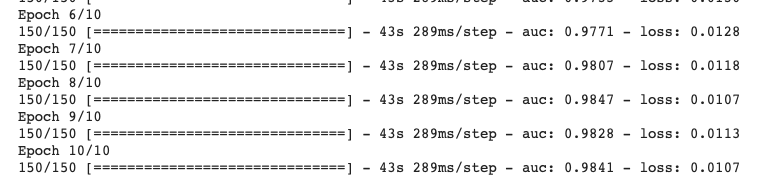### Predictions on Testset

We will now make predictions on the test dataset.

``````test['toxic'] = model.predict(test_data, verbose=1)
``````

Save the output to a CSV file and examine its contents.

``````test.to_csv('test.csv', index=False)
``````

The output is: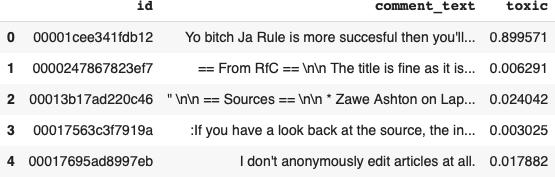The first record shows a toxicity of 89.95%, while the rest show a meager toxicity value. These values indicate that the first record contains the toxic contents while the rest are clear of slang words. Instead of probability value, we can print the output as toxic or non-toxic by providing the following trivial function:

``````def replace(toxic):
if toxic >=0.5:
toxic=1
else:
toxic=0

test['prediction']=test['toxic'].apply(lambda x : replace(x))
``````

The new output is as follows, where the prediction values are either 0 or 1.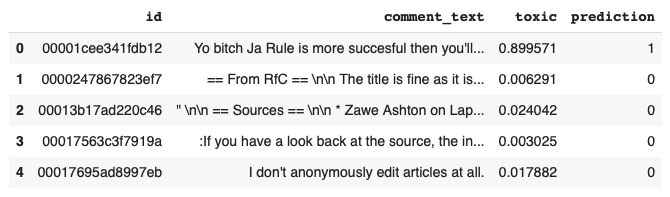We can also get the plot of how many toxic texts were detected in the test dataset by using the following code:

``````test.prediction.value_counts().plot(kind='bar')
plt.xlabel('toxic or non-toxic')
plt.ylabel('count')
``````

This is the output: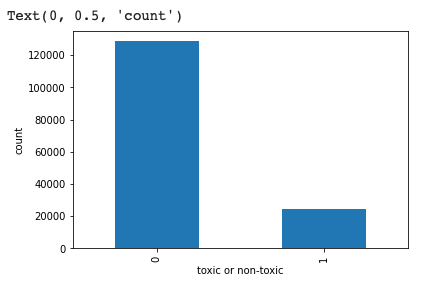## Predicting on Unseen Data

I will now show you how to predict toxicity in an unseen text passage. Note that we need to encode the passage text before feeding it to our model. I will demonstrate this process by first taking some text from our test dataset and then repeating the entire process for unseen data taken from Google search.

Let us pick up a text passage from the index value of 186 (randomly chosen) from our test dataset.

``````text1=test.comment_text
text1
``````

The text passage is as follows:
`'Are you also suggesting all paintings of black moors are fake too? are you saying that they should be white?'`
To encode this text, we write our encoding function as follows:

``````def fast_encode(texts, tokenizer, chunk_size=256, maxlen=512):
tokenizer.enable_truncation(max_length=maxlen)
all_ids = []

encs = tokenizer.encode_batch(texts)
all_ids.extend([enc.ids for enc in encs])

return np.array(all_ids)
``````

We do the prediction and print it using the following code:

``````p1=fast_encode([text1], fast_tokenizer, maxlen=512)
p1 = model.predict(p1)
if (replace(p1) == 0):
print ("Okay contents")
else:
print ("Contents not permitted")
``````

Try it on one more passage from the test dataset.

``````text2=test.comment_text
text2
``````

This is the passage:

`'Yo bitch Ja Rule is more succesful then you'll ever be whats up with you and hating you sad mofuckas...i should bitch slap ur pethedic white faces and get you to kiss my ass you guys sicken me. Ja rule is about pride in da music man. dont diss that shit on him. and nothin is wrong bein like tupac he was a brother too...fuckin white boys get things right next time.,'`

Do the prediction using the following code:

``````p2=fast_encode([text2], fast_tokenizer, maxlen=512)
p2=model.predict(p2)
if (replace(p2) == 0):
print ("Okay contents")
else:
print ("Contents not permitted")
``````

This is the output:
`Contents not permitted`
Finally, let us check the model's prediction on some random comments collected from Google search. The comment’s text is shown below:

``````text3 =["Every once in a while, I get the urge. You know what I'm talking about, don't you? The urge for destruction. The urge to hurt, maim, kill. It's quite a thing to experience that urge, to let it wash over you, to give in to it. It's addictive. It's all-consuming. You lose yourself to it. It's quite, quite wonderful. I can feel it, even as I speak, tapping around the edges of my mind, trying to prise me open, slip its fingers in. And it would be so easy to let it happen. But we're all like that, aren't we? We're all barbarians at our core. We're all savage, murderous beasts. I know I am. I'm sure you are. The only difference between us, Mr. Prave, is how loudly we roar. I know I roar very loudly indeed. How about you. Do you think you can match me"]
``````

Encode the comment and do the prediction:

``````p3=fast_encode(text3, fast_tokenizer, maxlen=512)
p3=model.predict(p3)
if (replace(p2) == 0):
print ("Okay contents")
else:
print ("Contents not permitted")
``````

This is the output:
`Contents not permitted`
The model has predicted this as a toxic text.

## Model Improvements

We trained our model to predict if it contains a toxic word. The training dataset contains more than one type of slang - toxic, severe_toxic, obscene, threat, insult, and identity_hate. To check for the presence of these other slang types in the text, either you will need to develop a sequence-to-sequence (many-to-many) model or create six different instances of the above model to predict the individual slang types. You can then write a separate function to combine the probability distributions for all the outputs and conclude on a binary output whether the contents are toxic (slang) or non-toxic (acceptable material).

## Conclusion

In this tutorial, you learned how to use a pre-trained model like BERT to predict the toxicity in a given text. The BERT model encodes the given text using its several stacked Encoders. This encoded output is fed to a Dense neural network that provides a binary representation of whether the input is toxic or non-toxic.